Aegidius
Plüssin tutorials/apps
Use the asterisk (*) character for wildcard searches
Searches on more than one word will be treated "as a phrase"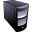Server Status
www.aplu.ch
www.java-online.eu
Print Text
© 2019, V10.1Java exemplarisch (Textbook, in German)Programming Concepts in Python Tutorial and Exercises in German, French and EnglishTCPCom An event-driven socket libarry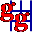JGameGrid        (Java Game Framework)JCardGame (JGameGrid Card Game Addon) Ported to AndroidJDroidLib     NEW: Support for Smartphone IDE (JGameGrid/TcpJLib for Android)JTurtleLib     (Java Turtle Graphics for Android)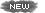Python-Exemplary Raspberry Pi Tutorial (in English and German)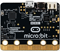New micro:bit     Single-board Computer Python Tutorial (Now released!)BrickPiLib Library for the BrickPi KitRaspiBrick Low-Budget Robotics with the Raspberry Pi EV3JLib (Library for Lego EV3 Robot) Support for Jython & AndroidTcpJLib (TCP/IP Communication)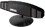KinectJLib (Kinect Controller Library for Java)JAW       (Java API Wrapper, 32/64bit)Python/Jython (Using TigerJython IDE)Java Utilities  (Graphics, Turtle)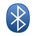Bluetooth Lib (For J2ME/J2SE)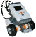NxtJLib (Library for Lego NXT Robot) Support for J2SE/J2ME & AndroidXboxController (Xbox Gamepad Library)Simulations    (for Physics Teachers)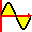Overtone           (Spectral Composition/Analysis)FtController  (Fischertechnik Java Library) CoverSheet      (Presentation Tool) Gidlet      (Java ME Framework) MidPak      (MIDlet Packaging) JavaOC      (Java Object Creator) JawGadget (Transparent Windows) C++/Champ      (Graphics Framework) Contact Home
JDroidLibThe source code of all examples is included in the JDroidLib distribution.

## Android Gesture DetectionThe Fling Gesture

Smartphone and tablet users often perform a "sweep" gesture by moving rapidly with one finger over the screen. The system's reaction depends on the direction and the speed of the sweep. With Android this action is called a "fling gesture" and is well supported by the Android API. (Keep in mind that if you put your finger on the screen, start moving it very slowly and then remove your finger gently, the gesture won't be count as a fling gesture.) As with touch events we implement the detection of a fling gesture as an event that triggers a callback method flingEvent(Point start, Point end, GGVector velocity) declared in a GGFlingListener interface. The callback reports the point where the finger first touched the screen, the point where the finger released the screen and a vector that is a proportional to the velocity of the movement between the two points. A pixel based coordinate system is used that has its origin in the upper left vertex of the screen (not the game grid window), the positive x axis is downward and the positive y axis to the right. We use toLocation() to convert coordinates returned from flingEvent() to pixel coordinates of the game grid window. In the first example you throw a tennis ball with a fling gesture. It then moves on a trajectory parabola like in the earth gravitational field. It's a basic situation used in many shooting games. From the class TennisBall you can learn how to make a physics simulation with GameGrid. TennisBall is-an Actor (is derived from Actor) and act() sets the state of the ball after one simulation period. We make a linear approximation and consider the simulation period as infinitesimal time step. The following design principles are applied:
• All position, velocity and acceleration variables are in real physical units (doubles, m, m/s, m/s^2)
• Some initial conditions are set by the constructor, but because the constructor is called before the actor is added to the game grid, some other parameters are unknown when the constructor runs, especially the actor's location and the simulation period. Because reset() is invoked when the actor is added to the game grid, these parameters can be easily retrieved here
• The state change from time t to t + dt is performed in act(). We use the well-known equations for a free falling object in the homogenous gravitational field
• The dimension factor dimFactor maps the screen dimension (integer pixel coordinates) to the real world dimensions
• When the actor leaves the game grid, it is removed to save memory and increase execution speed (especially important with colliding actors)
 package ch.aplu.tut; import ch.aplu.android.*; public class TennisBall extends Actor {   private final double g = 9.81; // in m/s^2   private double dimFactor;   private double x, y;  // in m    private double vx, vy; // in  m/s   private double dt;  // in s   public TennisBall(double vx, double vy, double dimFactor)   {     super("tennisball");     // Initial condition     this.vx = vx;     this.vy = vy;      this.dimFactor = dimFactor;   }      public void reset()   {     // Initial condition     x = getX() / dimFactor;     y = getY() / dimFactor;     dt = gameGrid.getSimulationPeriod() / 1000.0;  // in s   }      public void act()   {     vy = vy + g * dt;     x = x + vx * dt;     y = y + vy * dt;     setLocation(new Location(dimFactor * x, dimFactor * y));     if (!isInGrid())       removeSelf();   } }

For the purpose of a demonstration the application program is kept simple. We create a full-screen window in landscape orientation with a status bar, where we want to display the velocity values from the fling event (in a suitable decimal format). The velocity reported from the fling event needs to be scaled with vFactor.

 package ch.aplu.tut; import ch.aplu.android.*; import android.graphics.Point; import java.text.DecimalFormat; public class Ex09 extends GameGrid  implements GGFlingListener {   private final double courtWidth = 23.77; // m   private double dimFactor;   private final double vFactor = 50.0;    private GGStatusBar status;      public Ex09()   {     super(WHITE, false, true, null); // full screen, white boarder     setScreenOrientation(LANDSCAPE);     status = addStatusBar(20);   }   public void main()   {     addFlingListener(this);     dimensionFactor = getNbHorzCells() / courtWidth;     setSimulationPeriod(50);     doRun();     status.setText("Fling the ball!");   }   public boolean flingEvent(Point start, Point end, GGVector velocity)   {     TennisBall tennisBall =  new TennisBall(             vFactor * velocity.x, vFactor * velocity.y, dimFactor);     DecimalFormat d =  new DecimalFormat("#.#");     String vx = d.format(velocity.x);     String vy = d.format(velocity.y);     String v = d.format(velocity.magnitude());     status.setText("(vx, vy) = ("  + vx +  ", "  + vy +  "); v = "  + v);     addActorNoRefresh(tennisBall, toLocation(end.x, end.y));     return true;   } }Download Ex09 app for installation on a smartphone

Create QR code to download Android app to your smartphone.

Download sources (Ex09.zip).

With the knowledge of the above example it is not difficult to simulate the movement of a tennis ball subject to air friction. We assume that the frictional force is proportional to the square of the magnitude of the velocity and has a direction opposite to the velocity vector. To solve the problem it is highly recommended to use a vector notation and the GGVector class. Below are summarized the algebraic formulas and their implementation in Java.

 Quantity Mathematical notation Program implementation ForceGGVector f = g.mult(m).add(v.mult(-R * v.magnitude())); Acceleration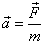GGVector a = f.mult(1 / m); New velocityGGVector vnew = v.add(a.mult(dt)); New position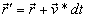GGVector rnew = r.add(v.mult(dt));

Using the vector notation the code of the modified class TennisBall1 that takes into account the air friction is clean and simple. The only modification in the application class is to replace TennisBall by TennisBall1.

 package ch.aplu.tut; import ch.aplu.android.*; import android.graphics.Point; public class TennisBall1 extends Actor {   private final GGVector g = new GGVector(0, 9.81);   private final double m = 0.057; // kg   private final double R = 0.005; // Air friction (N per (m/s)^2)   private double dimFactor;   private GGVector r;   private GGVector v;   private double dt;  // in s   public TennisBall1(GGVector v, double dimFactor)   {     super("tennisball");     // Initial condition     this.v = v;     this.dimFactor = dimFactor;   }      public void reset()   {     // Initial condition     r = new GGVector(getX() / dimFactor, getY() / dimFactor);     dt = gameGrid.getSimulationPeriod() / 1000.0;  // in s   }      public void act()   {     GGVector f = g.mult(m).add(v.mult(-R * v.magnitude()));     GGVector a = f.mult(1 / m);     GGVector vnew = v.add(a.mult(dt));     GGVector rnew = r.add(v.mult(dt));     setLocation(new Location(toPix(rnew).x, toPix(rnew).y));     gameGrid.getBg().drawLine(toPix(r), toPix(rnew));     r = rnew;     v = vnew();          if (!isInGrid())       removeSelf();   }      private Point toPix(GGVector z)   {     return new Point((int)(dimFactor * z.x + 0.5),        (int)(dimFactor * z.y + 0.5));   } }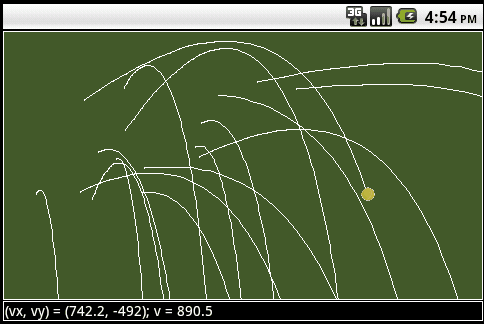Download Ex09a app for installation on a smartphone

Create QR code to download Android app to your smartphone.

Download sources (Ex09a.zip).

In the examples above, the gravitational field acceleration is supposed to be constant and directed from the top to the bottom of the application window. Because we use physical units, it is easy to modify the scenario to simulate a moving disk with air friction on the device surface (a rolling ball would behave like a disk with a somewhat greater mass). We use the internal acceleration sensor and initialize it by calling GGComboSensor.init() in the application class. In the modified Tennisball2.java we have just to add the two following lines at the beginning of act() instead of using a constant GGVector g:

double[] acc = Ex09b.sensor.getAcceleration(0);
GGVector g = new GGVector(-acc, acc);Download Ex09b app for installation on a smartphone

Create QR code to download Android app to your smartphone.

Download sources (Ex09b.zip).

### A prototype of a shooting game with fling

In many games using fling you select the object by tapping first the image you want to fling. This corresponds to the starting point of the fling event callback. In a typical "Shooting Gallery Game" the player launches selected balls to hit a moving figure. The following code shows several important implementation details:

• Ten balls are generated in createBalls(), shown at equal distances and inserted into a balls list. This list is used to define the collision partners for each figure created in createFigure(). All actors are added to the game grid using addActorNoRefresh() because the game grid is automatically refreshed by the simulation loop. This avoids the interference of refreshing by the simulation loop and callback methods that causes flickering.
• In the flingEvent() callback the coordinates of start and end must be converted to locations using the toLocation() method. If it is important to have only locations within the game grid, use toLocationInGrid(). end is used to limit the launch area to the lower part of the window. start selects the ball to launch by traversing the balls list and testing with isIntersecting(), if the start point is part of the non-transparent pixels of a particular ball. If this is the case, the ball's launch() method is invoked that starts the movement of the selected ball.
• When the figure and a ball collide, the collide() callback is invoked that removes the figure and hides the ball. (The ball is removed when it is outside the game grid). At this time the game score is updated.
• GameGrid.act() is the ideal place to put the code for a game supervisor, because act() is invoked in every simulation period. The supervisor checks if the game is over (because all balls are used). It also creates a new figure if the current figure has been hit (and removed).
 package ch.aplu.shootinggallery; import ch.aplu.android.*; import android.graphics.Point; import java.util.ArrayList; public class ShootingGallery extends GameGrid   implements GGFlingListener, GGActorCollisionListener {   private final int nbBalls = 10;   private GGStatusBar status;   private Ball[] balls = new Ball[nbBalls];   private int width, height;   private int nbPoints = 0;   private Figure f;   public ShootingGallery()   {     super(WHITE, false, true, windowZoom(1000));     setScreenOrientation(LANDSCAPE);     status = addStatusBar(30);   }   public void main()   {     width = getNbHorzCells();     height = getNbVertCells();     addFlingListener(this);     setSimulationPeriod(30);     getBg().setPaintColor(YELLOW);     getBg().drawLine(0, 2 * height / 10, width, 2 * height / 10);     getBg().setPaintColor(RED);     getBg().drawLine(0, height / 2, width, height / 2);     createBalls();     status.setText("Fling a ball!");     doRun();   }   public void act()   {     if (getNumberOfActors(Ball.class) == 0)  // Game over     {       status.setText("Game over. Total points: " + nbPoints);       f.removeSelf();       refresh();  // delay will inhibit automatic refresh       delay(3000);       createBalls();       createFigure();       nbPoints = 0;       status.setText("Fling a ball!");     }     if (getNumberOfActors(Figure.class) == 0)     {       delay(1000);       if (getNumberOfActors(Ball.class) != 0)         createFigure();     }   }   private void createBalls()   {     for (int i = 0; i < nbBalls; i++)     {       Ball ball = new Ball(this, (i + 1) * 10);       int d = width / nbBalls;       addActorNoRefresh(ball, new Location(d / 2 + i * d, height - d / 2));       balls[i] = ball;     }   }   private void createFigure()   {     f = new Figure();     double x = 10 + Math.random() * (width - 10);     addActorNoRefresh(f, new Location(x, height / 10),         (Math.random() < 0.5) ? 0 : 180);     f.setCollisionRectangle(new Point(0, 50), 50, 50);     for (Ball ball : balls)     {       if (ball != null)       {         f.addCollisionActor(ball);         f.addActorCollisionListener(this);       }     }   }   public boolean flingEvent(Point start, Point end, GGVector velocity)   {     Location endLocation = toLocationInGrid(end);     if (endLocation.y < height / 2)       return true;     Location startLocation = toLocationInGrid(start);     Point startPos = new Point(startLocation.x, startLocation.y);     int index = getBallIndex(startPos);     if (index != -1)     {       balls[index].launch(endLocation, velocity);       balls[index] = null; // tag as removed      }     return true;   }   // Returns the index of the ball cell where pt resides or   // -1 if pt is not part of any ball cell   private int getBallIndex(Point pt)   {     int d = width / nbBalls;     if (pt.y < height - d) // not in lower row       return -1;     int index = pt.x / d;     if (balls[index] == null) // tagged as removed       return -1;     return index;   }   public int collide(Actor actor1, Actor actor2)   {     actor1.removeSelf();     actor2.hide();     nbPoints += ((Ball)actor2).getValue();     displayResult();     return 10;   }   protected void displayResult()   {     status.setText("# point: " + nbPoints);   } }

A Ball is-an Actor (derived from Actor) and disables act() when created. Its launch() method uses the start and v parameter to set the starting location, the direction and the speed before enabling act() where the movement of the ball is engaged. In act() we just call move() using the speed value to move the ball to next position.

 package ch.aplu.shootinggallery; import android.graphics.Point; import ch.aplu.android.*; class Ball extends Actor {   private ShootingGallery app;   private final int speedFactor = 100;   private int speed;   private int value;   public Ball(ShootingGallery app, int value)   {     super("ball_" + value);     this.app = app;     this.value = value;     setActEnabled(false);   }      protected void launch(Location start, GGVector v)   {     setLocation(start);     setDirection(Math.toDegrees(v.getDirection()));     speed = (int)(speedFactor * v.magnitude());     setActEnabled(true);   }        protected int getValue()   {     return value;   }   public void act()   {     if (isInGrid())      move(speed);     else     {         app.displayResult();       removeSelf();     }   } }

Finally the Figure class is also derived from Actor and moves the figure forth and back.

 package ch.aplu.shootinggallery; import ch.aplu.android.*; public class Figure extends Actor {   public Figure()   {     super("figure_green");   }   public void act()   {     if (isMoveValid())       move((int)(10 * gameGrid.getZoomFactor()));     else       turn(180);   } }

It is up to your programming skills and your fantacy to include more challenge and thrill.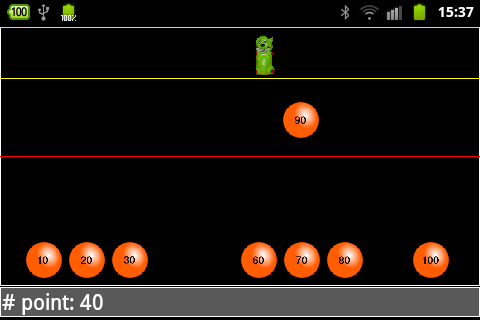Download ShootingGallery app for installation on a smartphone

Create QR code to download Android app to your smartphone.

Download sources (ShootingGallery.zip).

There are several alternatives to determine the object you want to fling. You may use Actor.contains():

 public boolean flingEvent(Point start, Point end, GGVector velocity) {   Location endLocation = toLocationInGrid(end);   if (endLocation.y < height / 2)     return true;   Location startLocation = toLocationInGrid(start);   Point startPos = new Point(startLocation.x, startLocation.y);   for (int i = 0; i < nbBalls; i++)   {     Ball ball = balls[i];     if (ball.contains(startPos))     {       ball.launch(endLocation, velocity);       balls[i] = null; // tag as removed        break;     }   }   return true; }

or GGCircle.isIntersecting():

 public boolean flingEvent(Point start, Point end, GGVector velocity) {   Location endLocation = toLocationInGrid(end);   if (endLocation.y < height / 2)     return true;   Location startLocation = toLocationInGrid(start);   Point startPos = new Point(startLocation.x, startLocation.y);   int d = width / nbBalls;   for (int i = 0; i < nbBalls; i++)   {     Ball ball = balls[i];     GGCircle circle =        new GGCircle(new GGVector(ball.getX(), ball.getY()), d / 2);     if (circle.isIntersecting(new GGVector(startPos)))     {         ball.launch(endLocation, velocity);       balls[i] = null; // tag as removed        break;     }     }   return true; }

or GGRectangle.isIntersecting():

 public boolean flingEvent(Point start, Point end, GGVector velocity) {   Location endLocation = toLocationInGrid(end);   if (endLocation.y < height / 2)     return true;   Location startLocation = toLocationInGrid(start);   Point startPos = new Point(startLocation.x, startLocation.y);   int d = width / nbBalls;   for (int i = 0; i < nbBalls; i++)   {     Ball ball = balls[i];     GGRectangle rectangle =       new GGRectangle(new GGVector(ball.getX(), ball.getY()), 0, d, d);     if (rectangle.isIntersecting(new GGVector(startPos), false))     {       ball.launch(endLocation, velocity);       balls[i] = null; // tag as removed        break;     }   }   return true; }Programming Android Smartphones Research Project PHBern TCP Socket LibraryTutorial Page 1 of 16 Tutorial Page 2 of 16 Tutorial Page 3 of 16 Tutorial Page 4 of 16 Tutorial Page 5 of 16 Tutorial Page 6 of 16 Tutorial Page 7 of 16 Tutorial Page 8 of 16 Tutorial Page 9 of 16 Tutorial Page 10 of 16 Tutorial Page 11 of 16 Tutorial Page 12 of 16 Tutorial Page 13 of 16 Tutorial Page 14 of 16 Tutorial Page 15 of 16Tutorial Page 16 of 16Android Apps 1 of 6 Android Apps 2 of 6 Android Apps 3 of 6 Android Apps 4 of 6 Android Apps 5 of 6 Android Apps 6 of 6 How to port JGameGrid programs to Android (and vice-versa)Support for Smartphone IDE (AIDE,...) Download ProjectBuilder App (Creates Projects for AIDE) Download ProjectBuilder for JavaSE (Creates Projects for Eclipse/Netbeans) Create QR code image(100x100 px JPEG) Install and ExecuteAdb Debug Console JDroidLib (+ TcpALib framework)RaspiBrick Robot Addon Free Download V 1.52 - Jul 17, 2017 Installation and Use How to develop AndroidApps under Linux(Ubuntu) ch.aplu.android JavaDoc JavaDoc ch.aplu.tcp JavaDoc JavaDoc JDroidLib Home Anleitung JDroidLib mit Eclipse unter Windows (in German) Anleitung JDroidLib mit Eclipse (allgemein)(in German) Anleitung JDroidLib mit Netbeans(in German) USB Driver forSamsung Mobile Phones(Windows 7, 32/64bit) FAQ/Forum